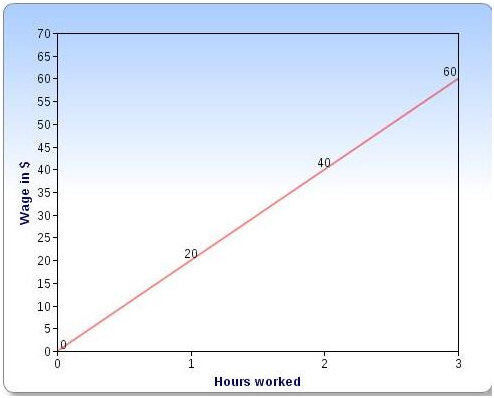# Linear Equations Graphing

## Online Tutoring Is The Easiest, Most Cost-Effective Way For Students To Get The Help They Need Whenever They Need It.

A pictorial representation which explains the relationship between x and y is called a graph for those variables. In case of a simultaneous linear equation in x and y the graph is a straight line. The set of points whose coordinates satisfy this equation will form the required straight line.

There are two methods for graphing

Method 1:

The equation in the standard form is written as y = m x + b where m is the slope of the line and b is the y intercept       which is a point on y axis.
♦ Y intercept is placed in the coordinate system
♦ Starting from y intercept and using the slope we locate one more point on the coordinate system.
♦ We draw a line between the y intercept and the other point.

Method 2:
♦ We can find the coordinates of any three points on the given line by giving arbitrary values to x and then using the         equation to find corresponding y values.
Then we plot the set of points on the graph

Example 1:

The points (3, -1) , (2, -3), ( 0 , -7) , (3.5 ,0 ) all lie on the graph of  2x – y = 7 because they satisfy the equation.

Example 2:John earns \$20 per hour. How much does he earn working 3 hours?
Draw a graph showing his wage.

Solution: Let   x be the number of hours worked ,y  be  wages
The equation is   y = 20x

 X ( hours worked) 0 1 2 3 Y ( wage in \$) 0 20 40 60